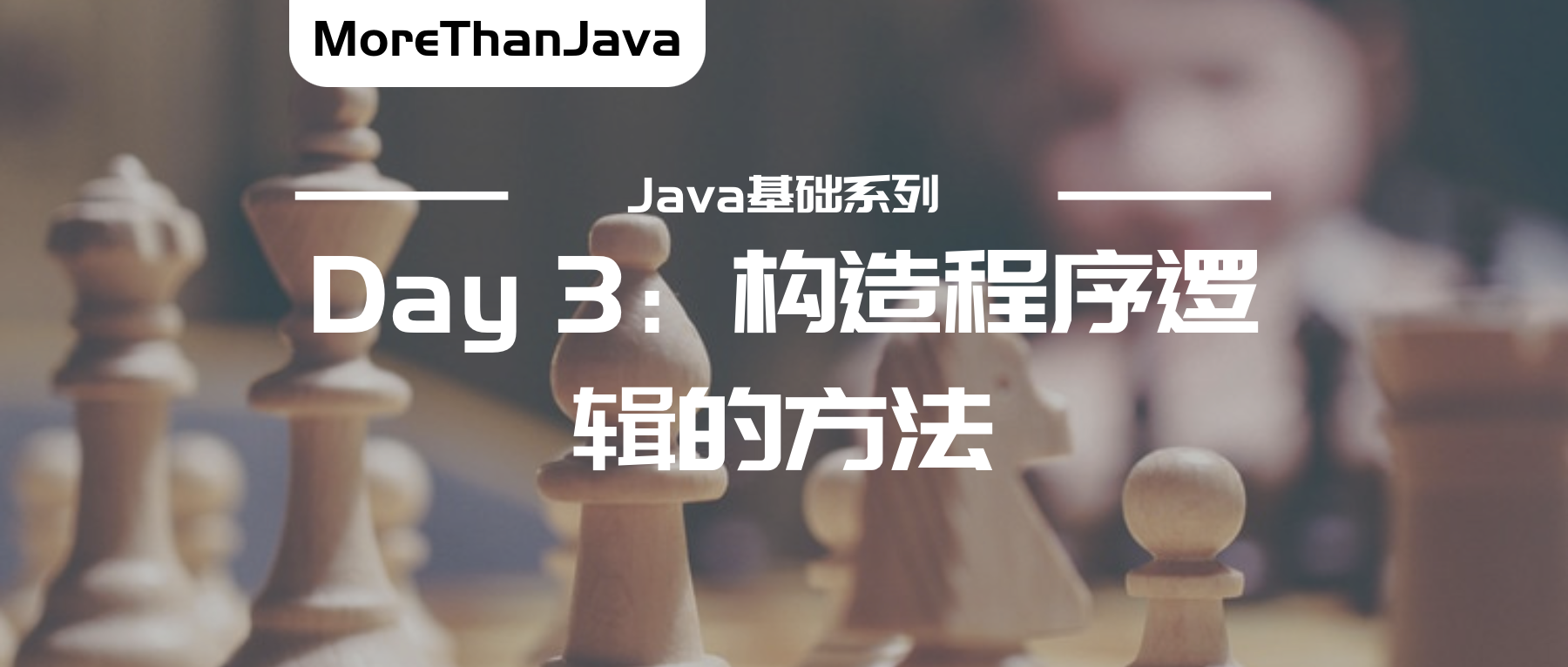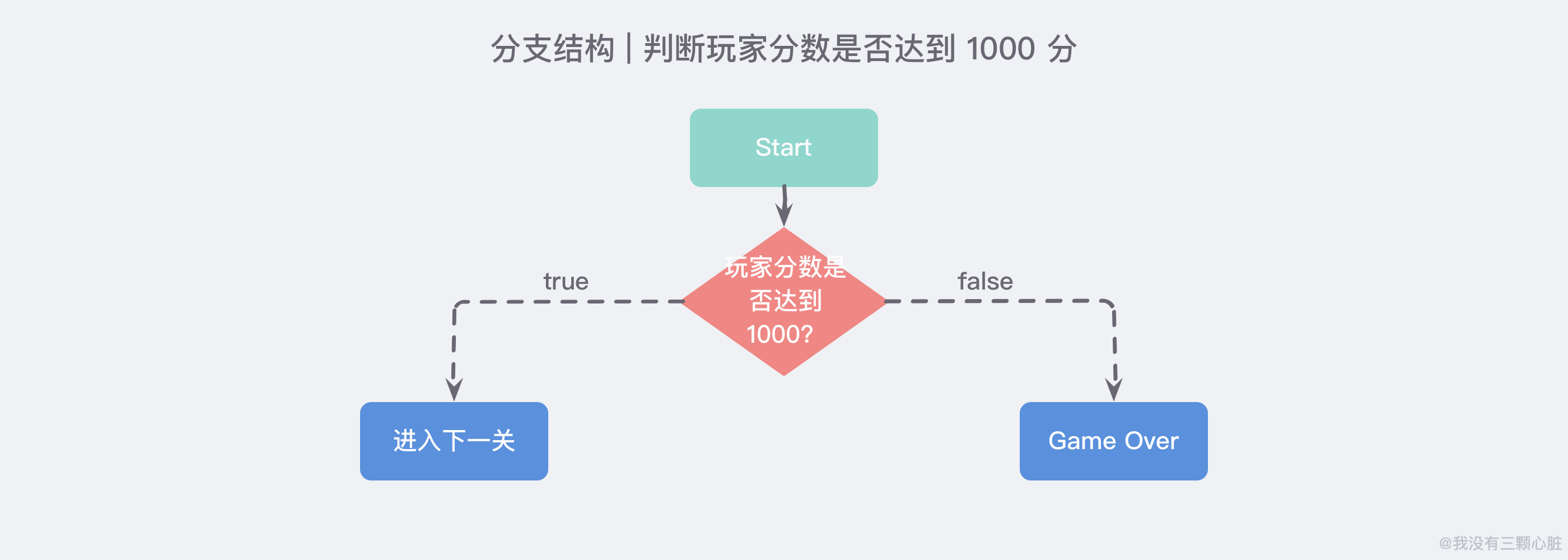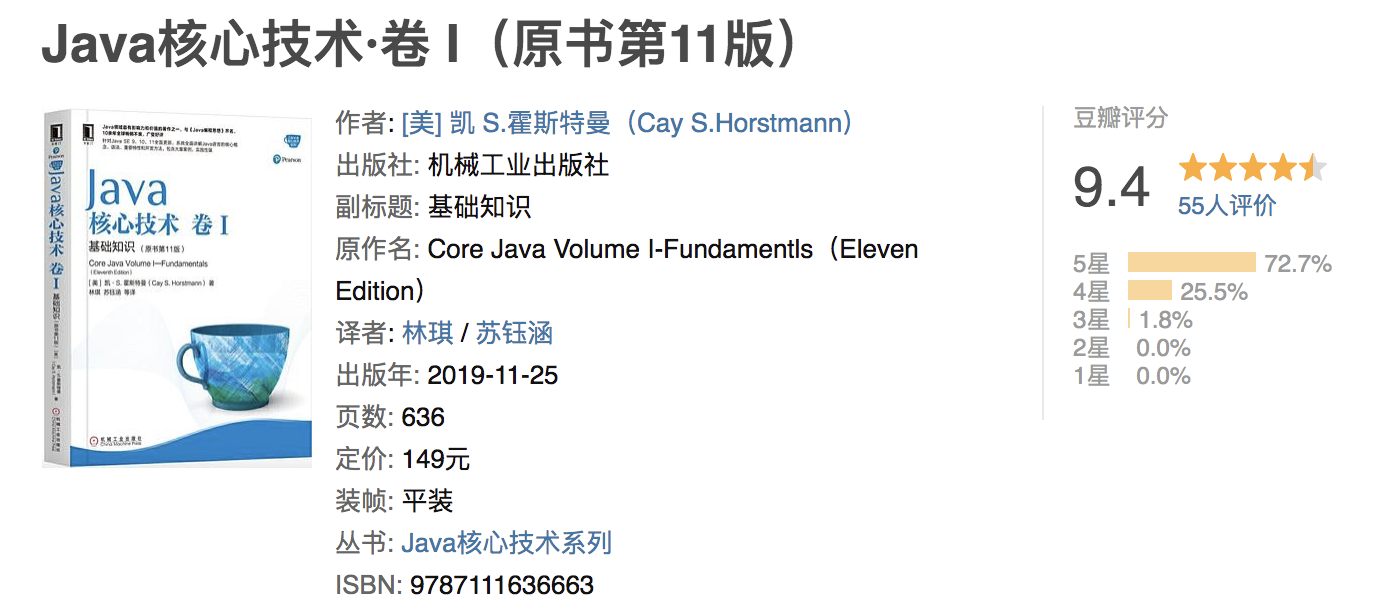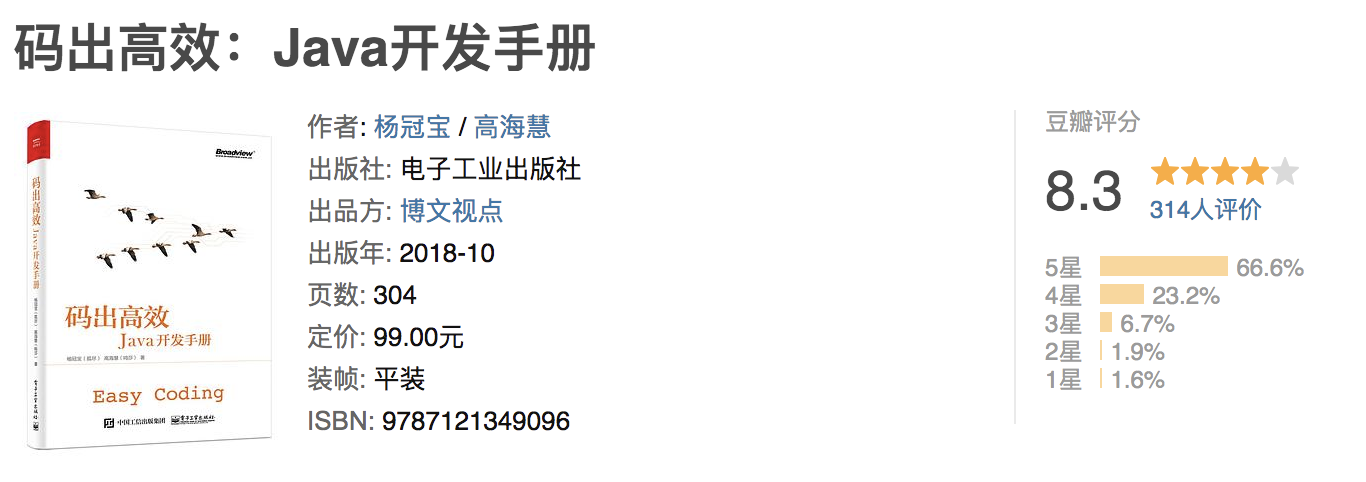# 「MoreThanJava」Day 3：构建程序逻辑的方法• 「MoreThanJava」 宣扬的是 「学习，不止 CODE」，本系列 Java 基础教程是自己在结合各方面的知识之后，对 Java 基础的一个总回顾，旨在 「帮助新朋友快速高质量的学习」
• 当然 不论新老朋友 我相信您都可以 从中获益。如果觉得 「不错」 的朋友，欢迎 「关注 + 留言 + 分享」，文末有完整的获取链接，您的支持是我前进的最大的动力！

# Part 1. 分支结构「是否进入下一关」这样的决策似乎很小，但是在编程中，复杂的决策是由许多这种小的决策组成的。下面是实现是否进入下一关的程序演示：

import java.util.Scanner;

public class Tester {

public static void main(String[] args) {
Scanner scan = new Scanner(System.in);

System.out.print("玩家是否达到 1000 分?(Y or N): ");

System.out.println("进入下一关");    // true branch
} else {
System.out.println("Game Over");   // false branch
}
}
}

System.out.print("玩家是否达到 1000 分?(Y or N): ");

answer = scan.nextLine();

if ("Y".equals(answer))

## if 条件语句

if 语句的基本语法是：

if (条件) {
// 条件满足时执行的语句
}

public class Tester {

public static void main(String[] args) {
int n = 70;
if (n >= 60) {
System.out.println("及格了");
}
System.out.println("END");
}
}

if (n >= 60)
System.out.println("及格了");

public class Tester {

public static void main(String[] args) {
int n = 50;
if (n >= 60)
System.out.println("及格了");
System.out.println("恭喜你"); // 注意这条语句不是if语句块的一部分
System.out.println("END");
}
}

## else 语句

if 语句还可以编写一个 else { ... }，当条件判断为 false 时，将执行 else 的语句块：

public class Tester {

public static void main(String[] args) {
int n = 70;
if (n >= 60) {
System.out.println("及格了");
} else {
System.out.println("挂科了");
}
System.out.println("END");
}
}

public class Tester {

public static void main(String[] args) {
int n = 70;
if (n >= 90) {
System.out.println("优秀");
} else if (n >= 60) {
System.out.println("及格了");
} else {
System.out.println("挂科了");
}
System.out.println("END");
}
}

if (n >= 90) {
// n >= 90为true:
System.out.println("优秀");
} else {
// n >= 90为false:
if (n >= 60) {
// n >= 60为true:
System.out.println("及格了");
} else {
// n >= 60为false:
System.out.println("挂科了");
}
}

### 注意顺序和临界条件

public class Tester {

public static void main(String[] args) {
int n = 100;
if (n >= 60) {
System.out.println("及格了");
} else if (n >= 90) {
System.out.println("优秀");
} else {
System.out.println("挂科了");
}
}
}

// 从大到小依次判断：
if (n >= 90) {
// ...
} else if (n >= 60) {
// ...
} else {
// ...
}

// 从小到大依次判断：
if (n < 60) {
// ...
} else if (n < 90) {
// ...
} else {
// ...
}

public class Tester {

public static void main(String[] args) {
int n = 90;
if (n > 90) {
System.out.println("优秀");
} else if (n >= 60) {
System.out.println("及格了");
} else {
System.out.println("挂科了");
}
}
}

# Part 2. 循环结构Java 中有三种主要的循环结构：

• while 循环；
• do...while 循环；
• for 循环 (在 Java 5 中还引入了一种主要用于数组的增强型 for 循环)

## while 循环

while 是最基本的循环，它的结构为：

while ( 布尔表达式 ) {
// 循环内容
}

### 实例

public class Test {

public static void main(String args[]) {
int x = 10;
while (x < 20) {
System.out.println("value of x : " + x);
x++;
}
}
}

value of x : 10
value of x : 11
value of x : 12
value of x : 13
value of x : 14
value of x : 15
value of x : 16
value of x : 17
value of x : 18
value of x : 19

## do…while 循环

do…while 循环和 while 循环相似，不同的是，do…while 循环至少会执行一次。

do {
// 代码语句
} while (布尔表达式);

### 实例

public class Test {

public static void main(String args[]) {
int x = 10;

do {
System.out.println("value of x : " + x);
x++;
} while (x < 20);
}
}

value of x : 10
value of x : 11
value of x : 12
value of x : 13
value of x : 14
value of x : 15
value of x : 16
value of x : 17
value of x : 18
value of x : 19

## for 循环

for 循环执行的次数是在执行前就确定的。语法格式如下：

for(初始化; 布尔表达式; 更新) {
// 代码语句
}

• 最先执行初始化步骤。可以声明一种类型，但可初始化一个或多个循环控制变量，也可以是空语句。
• 然后，检测布尔表达式的值。如果为 true，循环体被执行。如果为false，循环终止，开始执行循环体后面的语句。
• 执行一次循环后，更新循环控制变量。
• 再次检测布尔表达式。循环执行上面的过程。

### 实例

public class Test {

public static void main(String args[]) {

for (int x = 10; x < 20; x = x + 1) {
System.out.println("value of x : " + x);
}
}
}

value of x : 10
value of x : 11
value of x : 12
value of x : 13
value of x : 14
value of x : 15
value of x : 16
value of x : 17
value of x : 18
value of x : 19

## 控制循环

### break 关键字

break 主要用在循环语句或者 switch 语句中，用来跳出整个语句块。

break 跳出最里层的循环，并且继续执行该循环下面的语句。

#### 实例

public class Test {

public static void main(String args[]) {
int[] numbers = {10, 20, 30, 40, 50};

for (int x : numbers) {
// x 等于 30 时跳出循环
if (x == 30) {
break;
}
System.out.print(x);
System.out.print("\n");
}
}
}

10
20

### continue 关键字

continue 适用于任何循环控制结构中。作用是让程序立刻跳转到下一次循环的迭代。

for 循环中，continue 语句使程序立即跳转到更新语句。

while 或者 do…while 循环中，程序立即跳转到布尔表达式的判断语句。

#### 实例

public class Tester {

public static void main(String args[]) {
int[] numbers = {10, 20, 30, 40, 50};

for (int x : numbers) {
if (x == 30) {
continue;
}
System.out.print(x);
System.out.print("\n");
}
}
}

10
20
40
50

# Part 3. 构造程序逻辑## 经典的例子

### 题目一：寻找水仙花数

public class Tester {

public static void main(String[] args) {
findAllDaffodilNumberAndPrint();
}

/**
* 查找所有的水仙花数并打印
*/
public static void findAllDaffodilNumberAndPrint() {
for (int num = 100; num < 1000; num++) {
int low = num % 10;
int mid = num / 10 % 10;
int high = num / 100;
// Math.pow(x, 3) 相当于求 x 的 3 次方
if (num == Math.pow(low, 3) + Math.pow(mid, 3) + Math.pow(high, 3)) {
System.out.println(num);
}
}
}
}

### 题目二：百钱百鸡问题

public class Tester {

public static void main(String[] args) {
getResultAndPrint();
}

/**
* 获取百钱百鸡的结果并输出
*/
public static void getResultAndPrint() {
for (int cockNum = 0; cockNum < 20; cockNum++) {
for (int henNum = 0; henNum < 33; henNum++) {
int chickNum = 100 - cockNum - henNum;
if (5 * cockNum + 3 * henNum + chickNum / 3 == 100) {
System.out
.println("公鸡：" + cockNum + "只, 母鸡：" + henNum + "只, 小鸡：" + chickNum + "只");
}
}
}
}
}

# 要点回顾

1. 分支结构 ifelse 的使用和实例；
2. 循环结构 whiledo...whilefor 循环的使用和实例；
3. 控制循环的 breakcontinue 实例；
4. 构建程序逻辑的练习；

# 练习

## 练习 1：百分之成绩转换成等级制成绩

1. 如果输入成绩在 90 分以上 (含 90 分) 输出 A
2. 80 ~ 90(不含 90) 输出 B
3. 70 ~ 80(不含 80) 输出 C
4. 60 ~ 70(不含 70) 输出 D
5. 60 分以下输出 E

import java.util.Scanner;

public class Tester {

public static void main(String[] args) {
Scanner scan = new Scanner(System.in);

int score = scan.nextInt();
if (score >= 90) {
System.out.println("A");
} else if (score >= 80) {
System.out.println("B");
} else if (score >= 70) {
System.out.println("C");
} else if (score >= 60) {
System.out.println("D");
} else {
System.out.println("E");
}
}
}

## 练习 2：输入三条边长，如果能构成三角形就计算周长和面积

import java.util.Scanner;

public class Tester {

public static void main(String[] args) {
Scanner scan = new Scanner(System.in);

double a = scan.nextDouble();
double b = scan.nextDouble();
double c = scan.nextDouble();

if (a + b > c && a + c > b && b + c > a) {
double perimeter = a + b + c;
System.out.println("三角形周长为：" + perimeter);

double p = (a + b + c) / 2;
double area = Math.sqrt(p * (p - a) * (p - b) * (p - c));
System.out.println("三角形面积为：" + area);
} else {
System.out.println("不能构成三角形！");
}

}
}

## 练习 3：打印如下所示的三角形图案

*
**
***
****
*****
    *
**
***
****
*****
    *
***
*****
*******
*********

import java.util.Scanner;

public class Tester {

public static void main(String[] args) {
System.out.println("请输入行数：");

Scanner scanner = new Scanner(System.in);
int row = scanner.nextInt();

for (int i = 0; i < row; i++) {
for (int j = row - i - 1; j < row; j++) {
System.out.print("*");
}
// 换行
System.out.println();
}

for (int i = 0; i < row; i++) {
for (int j = 0; j < row; j++) {
if (j < row - i - 1) {
System.out.print(" ");
} else {
System.out.print("*");
}
}
// 换行
System.out.println();
}

for (int i = 0; i < row; i++) {
for (int j = 0; j < row - i - 1; j++) {
System.out.print(" ");
}
for (int j = 0; j < 2 * i + 1; j++) {
System.out.print("*");
}
// 换行
System.out.println();
}
}
}

# 自取资料

## 优秀入门资料选取

1. Introduction to Computer Science using Java - http://programmedlessons.org/Java9/index.html
2. Java零基础入门教程包含面向对象 - https://study.163.com/course/courseMain.htm?courseId=1003108028
3. 网易云课堂 - 顶尖中文大学计算机专业课程体系 - https://study.163.com/curricula/cs.htm
4. TeachYourselfCS-CN (自学计算机科学) - https://github.com/keithnull/TeachYourselfCS-CN
5. C语言中文网 Java 入门系列教程 - http://c.biancheng.net/java/10/
6. 廖雪峰 Java 教程 - https://www.liaoxuefeng.com/wiki/1252599548343744
7. 注重动手能力的 Java 教程 - https://how2j.cn/

## 推荐书籍

#### Java 核心技术·卷 I(原书第 11 版)#### 码出高效：Java开发手册# 参考资料

1. 《Java 核心技术 卷I》(第11版)
2. Introduction to Computer Science using Java - http://programmedlessons.org/Java9/index.html#part02
3. 菜鸟教程 - https://www.runoob.com/java/
4. C语言中文网 Java 入门系列教程 - http://c.biancheng.net/java/10/
5. Python 100 天从新手到大师 - https://github.com/jackfrued/Python-100-Days
• 本文已收录至我的 Github 程序员成长系列 【More Than Java】，学习，不止 Code，欢迎 star：https://github.com/wmyskxz/MoreThanJava
• 个人公众号 ：wmyskxz，个人独立域名博客：wmyskxz.com，坚持原创输出，下方扫码关注，2020，与您共同成长！